# GRE Quantitative Reasoning: Exponents & Roots Chapter Exam

Exam Instructions:

Choose your answers to the questions and click 'Next' to see the next set of questions. You can skip questions if you would like and come back to them later with the yellow "Go To First Skipped Question" button. When you have completed the practice exam, a green submit button will appear. Click it to see your results. Good luck!

### Page 1

#### Question 1 1. Simplify the following expression.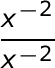#### Question 2 2. Which of the following radical expressions is equal to this exponential expression?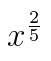#### Question 3 3. Simplify the following expression using positive exponents.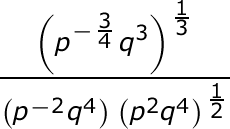### Page 2

#### Question 6 6. Simplify the expression below using only positive exponents.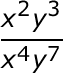#### Question 7 7. Solve the exponential equation below for x: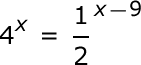#### Question 9 9. Simplify using ONLY positive exponents.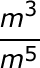#### Question 10 10. Which of the following is equivalent to the expression below?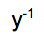### Page 3

#### Question 11 11. Solve the exponential equation below for x: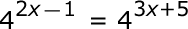#### Question 12 12. Which of the following is equivalent to the expression below?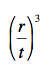#### Question 13 13. Simplify the expression using positive exponents.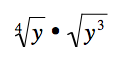#### Question 14 14. Solve, to 2 decimal places, the exponential equation below for x: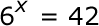#### Question 15 15. Which of the following is equivalent to the following expression?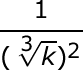### Page 4

#### Question 16 16. Which of the following is equivalent to the following expression?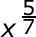#### Question 17 17. Which of the following is equivalent to the expression below?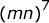#### Question 19 19. Which of the following would be true regarding the following exponential expression?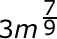#### Question 20 20. Simplify the expression below using positive exponents only.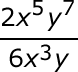### Page 5

#### Question 21 21. Using positive exponents, simplify the expression.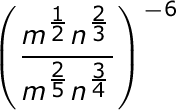#### Question 22 22. Which of the following exponential expressions is equal to this radical expression?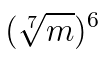#### Question 24 24. Simplify the following expression.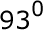#### Question 25 25. Simplify the following expression using positive exponents.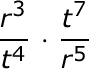### Page 6

#### Question 27 27. Which of the following is equivalent to the expression below?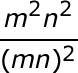#### Question 28 28. Simplify using ONLY positive exponents.#### Question 29 29. Simplify this expression using positive exponents.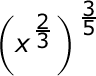#### Question 30 30. Simplify the following expression using positive exponents only.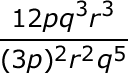#### GRE Quantitative Reasoning: Exponents & Roots Chapter Exam Instructions

Choose your answers to the questions and click 'Next' to see the next set of questions. You can skip questions if you would like and come back to them later with the yellow "Go To First Skipped Question" button. When you have completed the practice exam, a green submit button will appear. Click it to see your results. Good luck!

Support